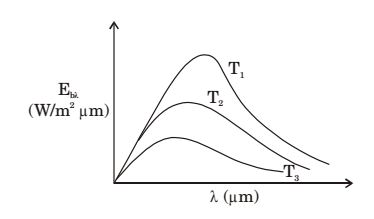## Heat Transfer Miscellaneous

#### Heat-Transfer

1. The radiative heat transfer rate per unit area (W/m2) between two plane parallel gray surfaces (emissivity = 0.9) maintained at 400 K and 300 K is (σ = Stefan Boltzmann constant = 5.67 × 10–8 W/m2K4)

1. For plane surfaces

 Q = σ(T14 - T24) A 1 + 1 - 1 ε1 ε2

Here , ε1 = ε2 = 0.9
 Q = 5.67 × 10-8(4004 - 3004) A 1 + 1 - 1 0.9 0.9

 = 8 ∥ .84 w ≈ 812 w m2 m2

##### Correct Option: B

For plane surfaces

 Q = σ(T14 - T24) A 1 + 1 - 1 ε1 ε2

Here , ε1 = ε2 = 0.9
 Q = 5.67 × 10-8(4004 - 3004) A 1 + 1 - 1 0.9 0.9

 = 8 ∥ .84 w ≈ 812 w m2 m2

1. For an opaque surface, the absorptivity (α), transmissivity (τ) and reflectivity ρ are related by the equation

1. α + τ + ρ = 1
for opaque surface, τ = 0
∴ α + ρ = 1

##### Correct Option: C

α + τ + ρ = 1
for opaque surface, τ = 0
∴ α + ρ = 1

1. The following figure was generated from experimental data relating spectral black body emissive power to wave length at the three temperatures T1, T2 and T3 (T1 > T2 >T3).The conclusion in that the measurements are

1. Wien's displacement law is not satisfied, i.e., λmax T = C
which tells lower λmax for higher temperature.

##### Correct Option: D

Wien's displacement law is not satisfied, i.e., λmax T = C
which tells lower λmax for higher temperature.

1. What is the value of the view factor for two inclined flat plates having common edge of equal length and wlth an angle of 20 degrees?

1.  F12 = F21 = 1 - sinθ2

 = 1 - sin20°= 0.826 2

##### Correct Option: A

 F12 = F21 = 1 - sinθ2

 = 1 - sin20°= 0.826 2

1. A diffuse radiation surface has